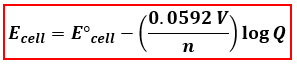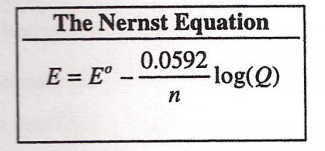# Problem: Consider this reaction.Sn2+ (aq) + 2 Fe3+ (aq) → Sn4+ (aq) + 2 Fe2+ (aq)     E° = 0.617 VWhat is the value of E when [Sn2+] and {Fe3+] are equal to 0.50 M and [Sn4+] and [Fe2+] are equal to 0.10 M?(A) 0.069V(B) 0.679 V(C) 0.658 V(D) 0.576

###### FREE Expert Solution
83% (388 ratings)
###### FREE Expert Solution

We are asked to find the cell potential of the given reaction. We will use the Nernst Equation to calculate for the cell potential with the given conditions. The Nernst Equation relates the concentrations of compounds and cell potential.Ecell = cell potential under non-standard conditions
cell = standard cell potential
n = number of e- transferred
Q= reaction quotient = [products]/[reactants]

Let’s first determine how many electrons were transferred in the reaction by separating the two half-reactions:

83% (388 ratings)###### Problem Details

Consider this reaction.

Sn2+ (aq) + 2 Fe3+ (aq) → Sn4+ (aq) + 2 Fe2+ (aq)     E° = 0.617 V

What is the value of E when [Sn2+] and {Fe3+] are equal to 0.50 M and [Sn4+] and [Fe2+] are equal to 0.10 M?

(A) 0.069V

(B) 0.679 V

(C) 0.658 V

(D) 0.576Frequently Asked Questions

What scientific concept do you need to know in order to solve this problem?

Our tutors have indicated that to solve this problem you will need to apply the The Nernst Equation concept. You can view video lessons to learn The Nernst Equation. Or if you need more The Nernst Equation practice, you can also practice The Nernst Equation practice problems.

What is the difficulty of this problem?

Our tutors rated the difficulty ofConsider this reaction.Sn2+ (aq) + 2 Fe3+ (aq) → Sn4+ (aq) +...as medium difficulty.

How long does this problem take to solve?

Our expert Chemistry tutor, Sabrina took 4 minutes and 30 seconds to solve this problem. You can follow their steps in the video explanation above.

What professor is this problem relevant for?

Based on our data, we think this problem is relevant for Professor N/A's class at Ryerson University.